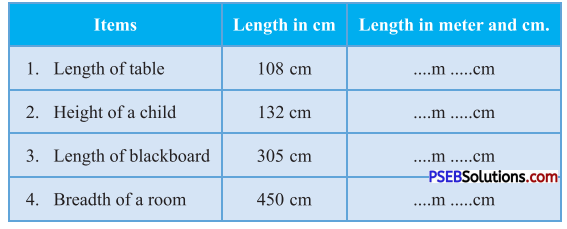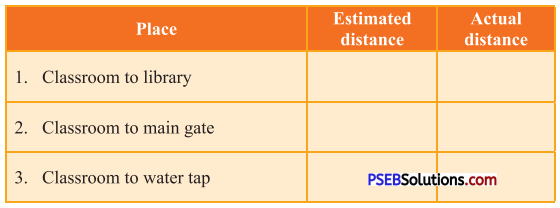# PSEB 4th Class Maths Solutions Chapter 5 Measurement Ex 5.3

Punjab State Board PSEB 4th Class Maths Book Solutions Chapter 5 Measurement Ex 5.3 Textbook Exercise Questions and Answers.

## PSEB Solutions for Class 4 Maths Chapter 5 Measurement Ex 5.3

(1 metre = 100 cm)

Question 1.
Convert into metre:
(a) 400 cm = ……. m
Solution:
4 m

(b) 700 cm = ……. m
Solution:
7 m

(c) 200 cm = …….. m
Solution:
2 m

(d) 800 cm = ……. m
Solution:
8 m

(e) 500 cm = ……….. m
Solution:
5 m

(f) 900 cm = …….. m
Solution:
9 in.

Question 2.
Convert into centimetres :
(a) 3 m = …… cm
Solution:
3 m = 3 × 100 cm
= 300 cm

(b) 6 m = …… cm
Solution:
6 m = 6 × 100 cm
= 600 cm

(c) 4 m = …… cm
Solution:
4 m = 4 × 100 cm
= 400 cm

(d) 9 m = ……. cm
Solution:
9 m = 9 × 100 cm
= 900 cm

(e) 2 m = …….. cm
Solution:
2 m = 2 × 100 cm
= 200 cm

(f) 5m = ……. cm
Solution:
5 m = 5 × 100 cm
= 500 cmQuestion 3.
Mohit measures length of given items with help of a 30 cm scale. Show this length in metre and centimetres.Solution:

1. 1 m 8 cm.
2. 1 m 32 cm.
3. 3 m 5 cm.
4. 4 m 50 cm.

Question 4.
Estimate the distance in metres and also find the actual distance with the help of metre rod or measurement tape.Solution:
Try yourself.